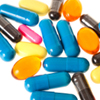# The logic of drug testing

John Haigh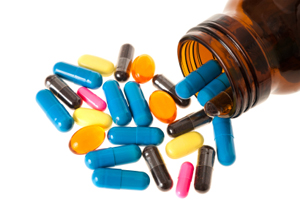How reliable are accusations of cheating based on drug tests?

In order to see whether an athlete has sought an unfair advantage using performance-enhancing drugs, various tests can be made. Their detail depends on what banned substance is being investigated, but the logic behind all such tests is identical: some "measurement" is made, and if its value exceeds some threshold, this is seen as sufficient evidence of cheating.

How likely is it that an athlete who fails the test really is a cheat, that is, how reliable is this accusation? In the language of probability, we seek the conditional probability that the athlete is guilty given they have failed the test, written as Pr(Guilty | Fail). Three numbers are required to work this out. The first is the so-called sensitivity of the test: the proportion of drug users who fail. In probability terms, this is the conditional probability that the athlete fails the test given they are guilty, Pr(Fail | Guilty). We would like this to be close to 100%. A second number is the test's specificity: the proportion of non-users who pass. Again, this should be close to 100%, meaning that Pr(Fail | Not Guilty) should be close to zero. The final quantity is the actual proportion of drug users in the relevant population, that is the group of athletes who might be tested. This is hard to know with precision, but we can make reasonable estimates.

If we have these three numbers we can use a mathematical result known as Bayes' theorem that gives us the answer (you can read more about Bayes' theorem on Plus). It turns out to be easier to work with odds, rather than probabilities. Recall that if the probability an event occurs is 80%, the probability it does not occur is 20%, so the odds on its occurrence are 80/20, or 4 to 1; if the chance is 90%, the odds are 90/10, or 9 to 1. Probabilities determine odds, and vice versa.

Before any evidence about drugs is sought, the probability that a randomly chosen athlete is a cheat is just the proportion of cheats in the population, so we use this figure to find the corresponding odds value. This ratio,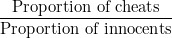is termed the prior odds of guilt.

Suppose an athlete fails the test. How should we find the posterior odds of guilt, that is the odds that they are a cheat, given the evidence of a failed test? First calculate the weight of evidence, defined as the ratio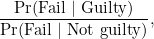using the sensitivity and specificity noted above. Now Bayes' theorem tells us that the answer we seek, the posterior odds, comes simply from multiplying the prior odds by this weight of evidence. You can then convert this to a probability, if you prefer.

Numbers often help. Suppose the proportion of cheats is 1%, the sensitivity is 95%, and the specificity is also 95%. Plainly, the prior odds of guilt are 1/99. The weight of evidence is 95/5 (agreed? Look carefully at its definition), so the posterior odds of guilt are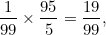about 0.19. To convert the odds into a probability, we divide the odds by 1 plus the odds: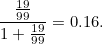This gives a probability of guilt of about 16% which, to most people, will be disappointingly low. Although the test gets things wrong only five times in a hundred among cheaters as well as innocents, it isn’t good enough. Could we really throw someone out of the Olympic Games on a 16% chance of being a cheat?

Despite the initially impressive numbers of 95% for the sensitivity and specificity of the test, to understand this unsatisfactory outcome, imagine a population of size 10,000, with 1% drug cheats. That means we have 9900 clean athletes, and 100 cheats. We expect the test to catch 95% of the cheats, that is 95 of them, but it will also finger 5% of the innocents, another 495 people. So 590 athletes fail the test, but only 95 of them — 16% of course — are genuine cheats.

We cannot expect any test to be 100% sensitive, or 100% specific. Mistakes will happen. Some authoritative body must set the thresholds, forming an acceptable balance between the mistake of accusing an innocent athlete of being a cheat, and the mistake of passing a drug user as clean. Knowing the sensitivity and specificity is not enough — a good idea of the size of the problem is required. And the fewer drug cheats there are, the better our tests must be to give a high enough chance of making the right decisions.

The following animation (from Understanding Uncertainty) illustrates our example. For simplicity it looks at a population of 100 and rounds the numbers involved to the nearest whole number. The "Testing" buttons shows you the outcome of a drug test on 100 people, assuming 1% of them are cheats and a test sensitivity and specificity of 95%. The "Trees" button shows you the result in the form of a tree diagram. The diagram shows that only 1 out of 6 — around 16% — of those who have tested positive have actually taken the drug.

You need to install the Adobe Flash Player to see the animation.